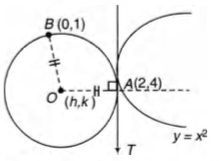# Q. The centre of the circle passing through the point (0, 1)and touching the curve $y = x^2 at (2,4)$ is

IIT JEEIIT JEE 1983Conic Sections

Solution:

## Let centre of circle be (h,k). so that $\hspace10mm OA^2 = OB^2$ $\Rightarrow \hspace5mm h^2 + (R - 1)^2 = (h - 2)^2 + (R - 4)^2$ $\Rightarrow \hspace5mm 4h + 6R - 19 = 0$ Also, slope of OA $= \frac {R - 4}{h - 2}$ 2 and slope of tangent at (2,4) to $y = x^2$ is 4. and (slope of OA) . (slope of tangent at A) = - 1 $\therefore \hspace10mm \frac{R-4}{h-2} . 4 = - 1$ $\Rightarrow \hspace10mm 4R - 16 = - h + 2$ $\hspace15mm h + 4R = 18 \, \, \, \, \, \, \, \, ...(ii)$ On solving Eqs. (i) and (ii), we get $\hspace20mm R = \frac {53}{10}$ and $\hspace16mm h = - \frac {16}{5}$ $\therefore Centre\, coordinates\, are \bigg( - \frac{16}{5}, \frac {53}{10} \bigg).$You must select option to get answer and solution

Integrals

Conic Sections

Conic Sections

AIEEE 2002 Sets

## 2. For $r = 0, 1, ... , 10,$ if $A_r,B_r$ and $C_r$ denote respectively the coefficient of $x^r$ in the expansions of $(1 + x)^{10}, (1 + x)^{20}$ and $(1 + x)^{30}$. Then, $\displaystyle \sum A_r(B_{10}B_r-C_{10}A_r)$ is equal to

IIT JEE 2010 Binomial Theorem

## 3. Let $\omega \ne 1$ be a cube root of unity and S be the set of all non-singular matrices of the form $\begin {bmatrix} 1 & a & b \\ \omega & 1 & c \\ \omega^2 & \omega & 1 \end {bmatrix}$ where each of a , b and c is either $\omega \ or \ omega^2$ Then, the number of distinct matrices in the set S is

IIT JEE 2011 Determinants

## 4. Perpendicular are drawn from points on the line $\frac{x+2}{2}=\frac{y+1}{-1}= \frac{z}{3}$ to the plane $x+ y + z = 3$. The feet of perpendiculars lie on the line

JEE Advanced 2013 Introduction to Three Dimensional Geometry

## 5. A ray of light along $x+\sqrt 3 \, y=\sqrt 3$ gets reflected upon reaching X-axis, the equation of the reflected ray is

JEE Main 2013 Straight Lines

## 6. A ray of light along $x+\sqrt 3 \, y=\sqrt 3$ gets reflected upon reaching X-axis, the equation of the reflected ray is

JEE Main 2013 Straight Lines

## 7. A ray of light along $x+\sqrt 3 \, y=\sqrt 3$ gets reflected upon reaching X-axis, the equation of the reflected ray is

JEE Main 2013 Straight Lines

## 8. Let AB be a chord of the circle $x^2 + y^2 = r^2$ subtending a right angle at the centre. Then, the locus of the centroid of the $\Delta PAB$ as P moves on the circle, is

IIT JEE 2001 Conic Sections